### Home > MC2 > Chapter 10 > Lesson 10.2.3 > Problem10-111

10-111.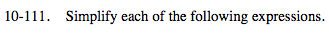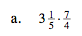Convert the mixed number into a fraction greater than one.

$\frac{16}{5}\left(\frac{7}{4}\right)$

$\frac{28}{5}=5\frac{3}{5}$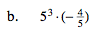Refer to the Math Notes box of Lesson 10.1.6.

Notice that the 5 in the denominator would have an exponent of 1.

5(3 − 1)(−4)

52(−4) = −100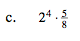This can be rewritten as:

$2^{4}\left(\frac{5}{2^{3}}\right)$

See part (b).

10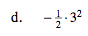Use the order of operations.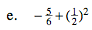Use the order of operations.

$-\frac{5}{6}+\frac{1}{4}$

$-\frac{20}{24}+\frac{6}{24}=-\frac{14}{24}$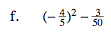Notice that squaring a negative number has the same result as multiplying two negative numbers: the product is always positive.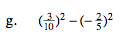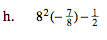$8^{(2-1)}(-7)-\frac{1}{2}$
$8(-7)-\frac{1}{2}$
$(-56)-\frac{1}{2}$
$-56\frac{1}{2}$